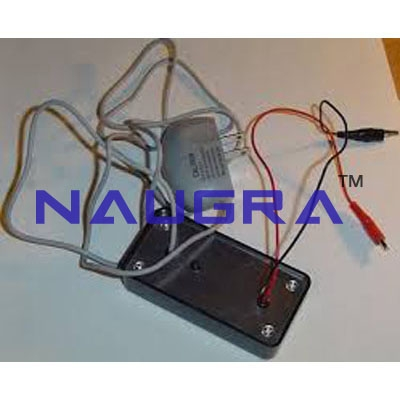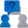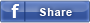• Enquiry# Measurement Of Low Resistances For Electrical Lab Training

CAT NO: DIDACTICNLE-Export-048002

### Description & Specification:

Measurement Of Low Resistances For Electrical Lab Training Workshop.
Principle The resistances of various DC conductors are determined by recording the current / voltage characteristic. The resistivity of metal rods and the contact resistance of connecting cords are calculated.   Tasks To plot the current / voltage characteristics of metal rods (copper and aluminium) and to calculate their resistivity. To determine the resistance of various connecting cords by plotting their current / voltage characteristics and calculating the contact resistances.   What you can learn about Ohm's law Resistivity Contact resistance Conductivity Four-wire method of measurementAdd to Wish List